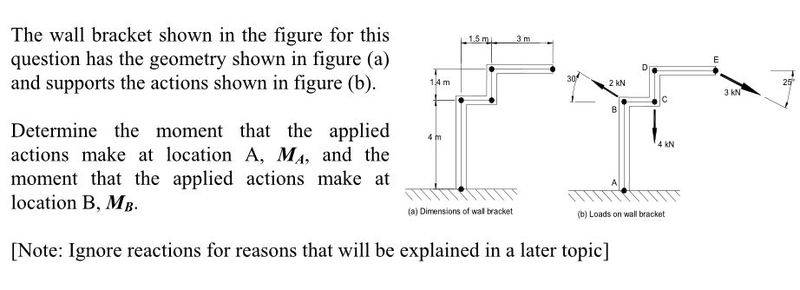# Moments about a point (statics)

## Homework StatementM = F*r

## The Attempt at a Solution

I tried splitting each force into its xy components and solve but the answer is wrong. Here is my attempt:

For point A:

Horizontal moments:

(3 * cos 25) * 5.4
- (2 * cos 30) * 4

Vertical moments:
- 4 * 1.5
- (3 sin 25) * 4.5

Then add them to determine the final moment and I get -3.9513 kNm but it's not the same as the correct answer which is -19.46 kNm.

What am I missing?

Last edited:

OK... it turns out I have to reverse the sign for the horizontal components so it becomes like this:

-(3 * cos 25) * 5.4
+(2 * cos 30) * 4

But I don't understand why... the 3 kNm force is pulling to the right hence its horizontal component is positive.

Can someone explain?

PhanthomJay
Homework Helper
Gold Member
OK... it turns out I have to reverse the sign for the horizontal components so it becomes like this:

-(3 * cos 25) * 5.4
+(2 * cos 30) * 4

But I don't understand why... the 3 kNm force is pulling to the right hence its horizontal component is positive.

Can someone explain?
The direction of the force should not be used when determining the direction (sign) of the moment about a point. What is important in determing the sign of the moment is determining whether the tendency of the force to rotate the object is clockwise (cw) or counterclockwise (ccw). Arbitrarily (or by convention) choosing ccw as plus (+), then cw is minus (-). Look at all the forces again and see if the tendency of the force components is to rotate the object cw or ccw about the chosen point. Then try solving for the moment of the forces about B.

Wecome to Physics Forums!